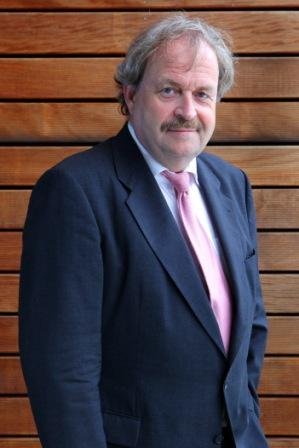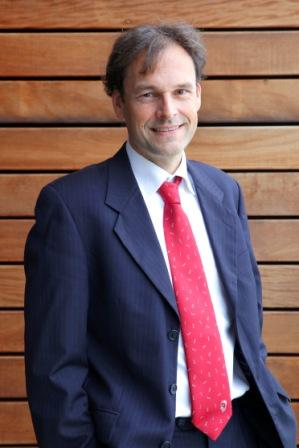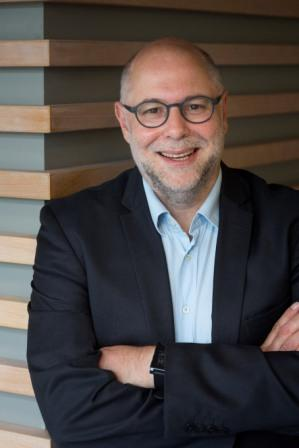# Large Deviations and Quantum Computing

On 30 May - 3 June 2016 NETWORKS organizes the third Training Week for PhD Students. The topics of this Training Week are Large Deviations and Quantum Computing. Harry Buhrman and Frank den Hollander are the main lecturers during this Training Week.

One of the exciting aspects of NETWORKS is that it covers stochastic and algorithmic  aspects of networks. This provides a unique opportunity to educate young researchers in both areas. To reach that goal, NETWORKS organizes Training Weeks. Each training week will combine two topics, one from stochastics and one from algorithmics. The training weeks will be held off-campus, so that they also serve as community-building activities.

## Target group

All NETWORKS PhD students are expected to participate in these Training Weeks, but other researchers from NETWORKS are very welcome as well. For participants of NETWORKS it is possible to subscribe against a fee of € 600,00. If you are interested please send an email to: info@thenetworkcenter.nl.

## Large deviationsThe lectures on large deviations are given by Frank den Hollander (Topics 1,2, and 4) and Michel Mandjes (Topic 3).

Large Deviation Theory is a part of probability theory that deals with the description of events where sums of random variables deviate from their mean by more than a 'normal' amount, i.e., beyond what is described by the central limit theorem. A precise calculation of the probabilities of such events turns out to be crucial for the study of expectations of exponential functionals of sums of random variables, which come up in a variety of different contexts. Large deviation theory finds application in probability theory, statistics, operations research, ergodic theory, information theory, statistical physics, complex networks, finance and insurance.

The goal of these lectures is to present the basics of large deviation theory and
to discuss two specific applications, one in rare-event simulation and one in statistical
physics.

## Topic 1: Overview of LD-theory, Part I

Background and motivation, Large Deviation Principle (LDP), Varadhan lemma and
Bryc lemma, Contraction principle, Exponential tilting.

## Topic 2: Overview of LD- theory, Part II

Dawson-Gärtner projective limit LDP, Cramèr theorem, Sanov theorem, Gärtner-Ellis theorem, Empirical process LDP.

## Topic 3: Rare-event simulation, importance sampling

Logarithmic vs. exact asymptotics, Bahadur-Rao theorem, Cramer-Lundberg theorem,
change-of-measure by exponential twisting, logarithmic efficiency, bounded relative error,
vanishing relative error, rare-event simulation in queueing networks.

## Topic 4: Breaking of ensemble equivalence for networks with topological constraints

Micro-canonical ensemble, Canonical ensemble, Relative entropy, Random graphs with a uni-partite, bi-partite or multi- partite structure, role of modularity and community structure.

## Quantum ComputingThe lectures on quantum computing are given by Harry Buhrman.

Today's computers---both in theory (Turing machines) and practice (PCs and smart phones)---are based on classical physics. However, modern quantum physics tells us that the world behaves quite differently. A quantum system can be in a superposition of many different states at the same time, and can exhibit interference effects during the course of its evolution. Moreover, spatially separated quantum systems may be entangled with each other and operations may have ``non-local'' effects because of this. Quantum computation is the field that investigates the computational power and other properties of computers based on quantum-mechanical principles. Its main building block is the qubit which, unlike classical bits, can take both values 0 and 1 at the same time, and hence affords a certain kind of parallelism. The laws of quantum mechanics constrain how we can perform computational operations on these qubits, and thus determine how efficiently we can solve a certain computational problem. Quantum computers generalize classical ones and hence are at least as efficient. However, the real aim is to find computational problems where a quantum computer is much more efficient than classical computers. For example, Peter Shor in 1994 found a quantum algorithm that can efficiently factor large integers into their prime factors. This problem is generally believed to take exponential time on even the best classical computers, and its assumed hardness forms the basis of much of modern cryptography (particularly the widespread RSA system). Shor's algorithm breaks all such cryptography. A second important quantum algorithm is Grover's search algorithm, which searches through an unordered search space quadratically faster than is possible classically. In addition to such algorithms, there is a plethora of other applications: quantum cryptography, quantum communication, simulation of physical systems, and many others.

## Location

Congrescentrum De Werelt • Westhofflaan 2 • 6741 KH Lunteren • www.dewerelt.nl

## Programme

Programme Training week 3

Events